AP State Syllabus AP Board 7th Class Maths Solutions Chapter 11 Exponents Ex 1 Textbook Questions and Answers.

## AP State Syllabus 7th Class Maths Solutions 11th Lesson Exponents Exercise 1Question 1.
Write the base and the exponent in each case. Also, write the term in the expanded form.
(i) 34
(ii) (7x)2
(iii) (5ab)3
(iv) (4y)5
Solution: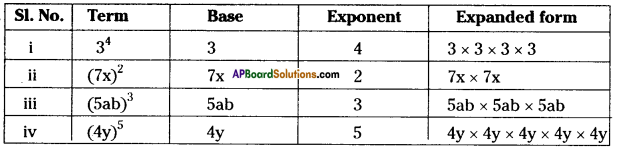Question 2.
Write the exponential form of each expression.
(i) 7 × 7 × 7 × 7 × 7
(ii) 3 × 3 × 3 × 5 × 5 × 5 × 5
(iii) 2 × 2 × 2 × 3 × 3 × 3 × 3 × 5 × 5 × 5
Solution:
(i) 7 × 7 × 7 × 7 × 7 = 75
(ii) 3 × 3 × 3 × 5 × 5 × 5 × 5 = 33 × 54
(iii) 2 × 2 × 2 × 3 × 3 × 3 × 3 × 5 × 5 × 5 = 23 × 34 × 53Question 3.
Express the following as the product of exponents through prime factorization.
(i) 288
(ii) 1250
(iii) 2250
(iv) 3600
(v) 2400
Solution:
(i) 288
1) 288 = 2 × 144
= 2 × 2 × 72
= 2 × 2 × 2 × 36
= 2 × 2 × 2 × 2 × 18
= 2 × 2 × 2 × 2 × 2 × 9
= (2 × 2 × 2 × 2× 2) × (3 × 3)
= 25 × 32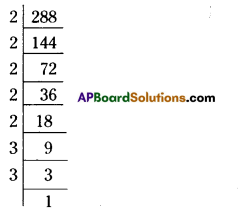(ii) 1250 = 2 × 625
= 2 × 5 × 125
= 2 × 5 × 5 × 25
= 2 × 5 × 5 × 5 × 5
= 2 × 54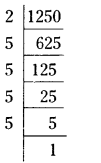(iii) 2250 = 2 × 1125
= 2 × 3 × 375
= 2 × 3 × 3 × 125
= 2 × 3 × 3 × 5 × 25
= 2 × 3 × 3 × 5 × 5 × 5
= 21 × 32 × 53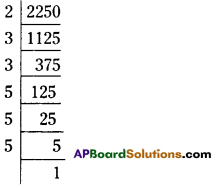(iv) 3600 = 2 × 1800
= 2 × 2 × 900
=2 × 2 × 2 × 450
=2 × 2 × 2 × 2 × 225
= ( 2 × 2 × 2 × 2 ) × 3 × 75
= (2 × 2 × 2 × 2) × (3 × 3) × 25
= (2 × 2 × 2 × 2) × (3 × 3) × (5 × 5)
= 24 × 32 × 52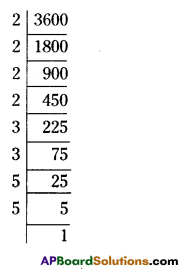(v) 2400
2400 = 2 × 1200
= 2 × 2 × 600
= 2 × 2 × 2 × 300
= 2 × 2 × 2 × 2 × 150
= 2 × 2 × 2 × 2 × 2 × 75
= (2 × 2 × 2 × 2 × 2) × 3 × 25
= (2 × 2 × 2 × 2 × 2) × 3 × (5 × 5)
= 25 × 31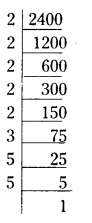Question 4.
Identify the greater number in each of the following pairs.
(i) 23 or 32
(ii) 53 or 35
(iii) 28 or 82
Solution:
(i) 23 or 32 = 23 = 2 × 2 × 2 = 8 and 32 = 3 × 3 = 9
∴ 23 < 32 or 32 > 23

(ii) 53 or 35 = 53 = 5 × 5 × 5 = 125 and 35 = 3 × 3 × 3 × 3 × 3 = 243
∴ 35 > 53(iii) 28 or 82 = 28 = 2 × 2 × 2 × 2 × 2 × 2 × 2 × 2 = 256
82 = 8 × 8 = 64
∴ 28 > 82

Question 5.
If a = 3, b = 2 find the value of
(i) ab + ba
(ii) aa + bb
(iii) (a + b)ab
(iv)(a – b)a
Solution:
(i) ab + ba = 32 + 23 = 3 × 3 + 2 × 2 × 2 = 9 + 8 =17
(ii) aa + bb = 33 + 22 = 3 × 3 × 3 + 2 × 2 = 27 + 4 = 31
(iii) (a + b)b = (3 + 2)2 = 52 = 5 × 5 = 25
(iv)(a – b)a = (3 – 2)2= 12 = 1 × 1 = 1 .